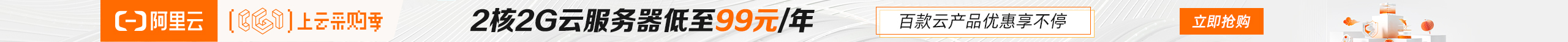# C博客作业05--指针

| 这个作业属于哪个班级 | C语言--网络2011/2012 |
| ---- | ---- | ---- |
| 这个作业的地址 | C博客作业05--指针 |
| 这个作业的目标 | 学习指针相关内容 |
| 姓名 | 李雷默 |

# 0.展示PTA总分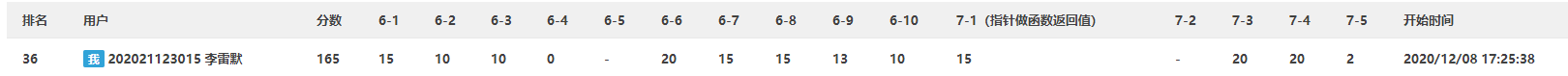# 1.本章学习总结

## 1.1 指针定义、指针相关运算、指针做函数参数。

### 1.1.2运算：

    int    a = 10,   *pa = &a, *paa = &a;
double b = 99.9, *pb = &b;
char   c = '@',  *pc = &c;
//最初的值
printf("&a=%s, &b=%s, &c=%s\n", &a, &b, &c);
printf("pa=%s, pb=%s, pc=%s\n", pa, pb, pc);
//加法运算
pa++; pb++; pc++;
printf("pa=%s, pb=%s, pc=%s\n", pa, pb, pc);
//减法运算
pa -= 2; pb -= 2; pc -= 2;
printf("pa=%s, pb=%s, pc=%s\n", pa, pb, pc);
//比较运算
if(pa == paa){
printf("%d\n", *paa);
}else{
printf("%d\n", *pa);
}


### 1.1.3指针做函数参数：

void swap(int *p1, int *p2){
int temp;
temp = *p1;
*p1 = *p2;
*p2 = temp;
}


## 1.2 字符指针:

   char *str = "qimobuguake";
int len = strlen(str), i;

//直接输出字符串
printf("%s\n", str);
//使用*(str+i)
for(i=0; i<len; i++){
printf("%c", *(str+i));
}
printf("\n");
//使用str[i]
for(i=0; i<len; i++){
printf("%c", str[i]);
}


## 1.3 指针做函数返回值：

C语言允许函数的返回值是一个指针，我们将这样的函数称为指针函数。用指针作为函数返回值时，函数运行结束后会销毁在它内部定义的所有局部数据，包括局部变量、局部数组和形式参数。

## 1.5 指针数组及其应用：

dataType *(arrayName[length]);


## 1.6 二级指针：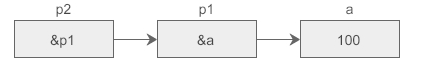• 可以使用&获取指针的地址。
• 每增加一级指针，在定义指针变量时就得增加一个星号*。

## 1.7 行指针、列指针：

int *(p1);  //指针数组，可以去掉括号直接写作 int *p1;
int (*p2);  //二维数组指针，不能去掉括号


# 2.PTA实验作业

## 2.1 7-3 字符串的冒泡排序

### 2.1.1 伪代码

if (strcmp(a[i], a[i + 1]) > 0)\\运用strcmp比较a[i]和a[i+1]大小，当a[i]>a[i+1]时，进行排序
{
strcpy(b, a[i]);       \\运用strcpy实现[i]和a[i+1]交换位置
strcpy(a[i], a[i + 1]);
strcpy(a[i + 1], b);
}


### 2.1.2 代码截图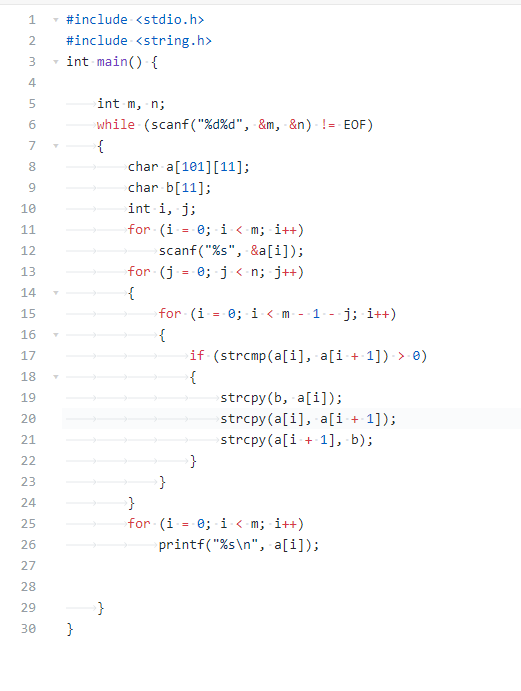## 2.2 6-9 合并两个有序数组（2）

### 2.2.1 伪代码

        for (i = 0; i < m; i++)//新建一个数组c将a、b放进c中
{
*c = a[i];
c++;
}
for (i = 0; i < n; i++)
{
*c = b[i];
c++;
}
for (i = 1; i < m + n; i++)//通过冒泡法排序升序排序数组
{
for (j = i + 1; j < m + n; j++) {
if (a[i] > a[j])
{
t = a[i];
a[i] = a[j];
a[j] = t;
}


### 2.2.2 代码截图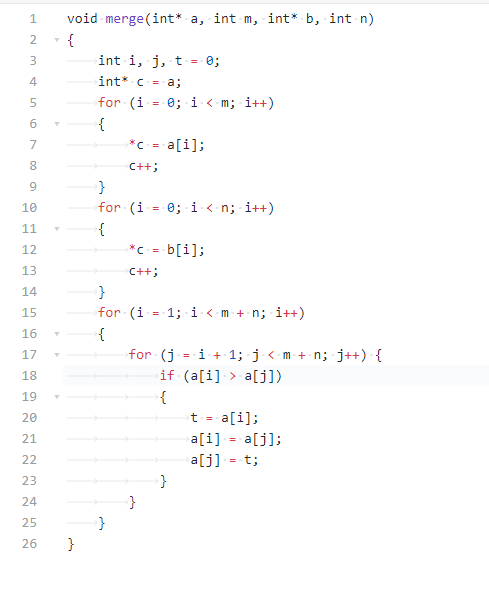### 2.2.3 找一份同学代码（尽量找思路和自己差距较大同学代码）比较，说明各自代码特点。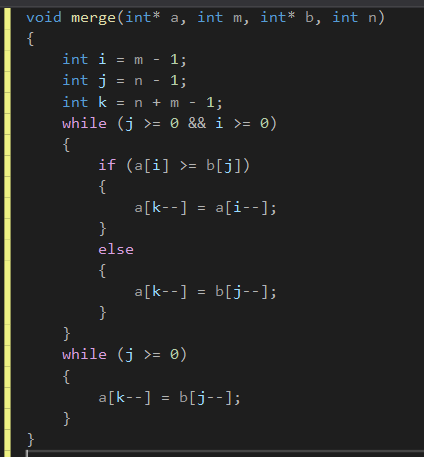## 2.3 7-4 说反话-加强版

### 2.3.1 伪代码

	for (i = len - 1; i >= 0; i--)\\逆向输入数据
{
if (a[i] != ' ' && a[i] != '\n')
{
tail = i;
for (j = i - 1; j >= 0; j--)
{
if (a[j] == ' ')
{
break;
}
if (j == 0)
{
break;
}
}
i = j;
if (count > 0)\\输出一个空格
{
printf(" ");
}
for (j = head; j <= tail; j++)\\输出数据
{
printf("%c", a[j]);
}


### 2.3.2 代码截图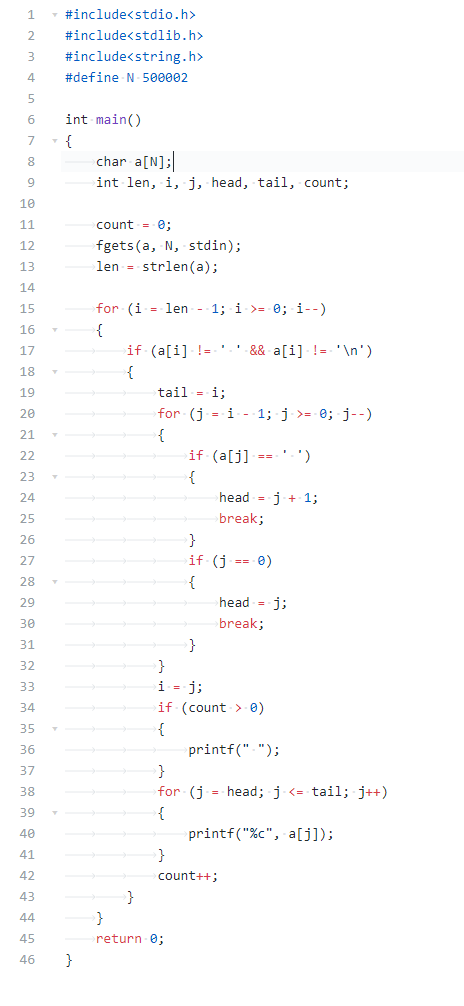### 2.3.3 请说明和超星视频做法区别，各自优缺点。

posted @ 2020-12-27 22:08  a丶落雨未  阅读(111)  评论(0编辑  收藏  举报